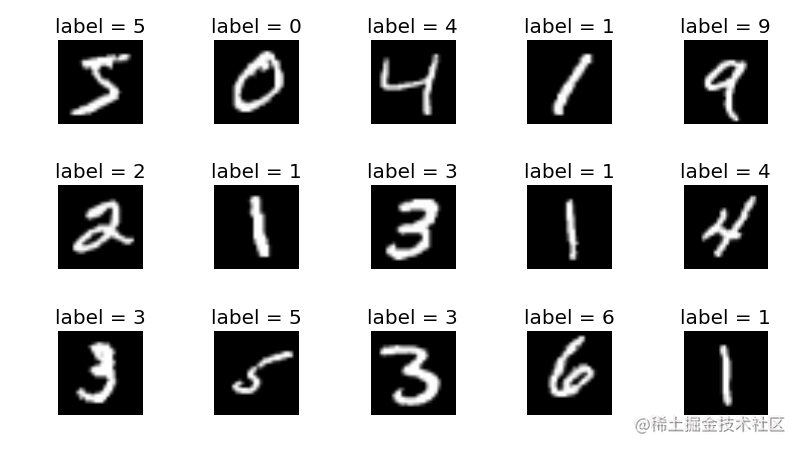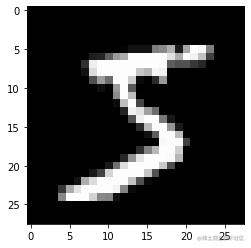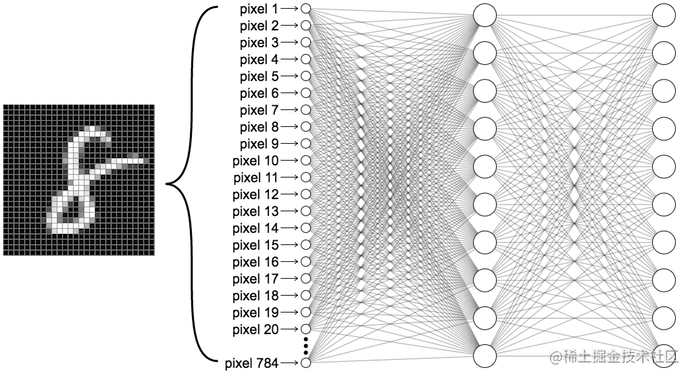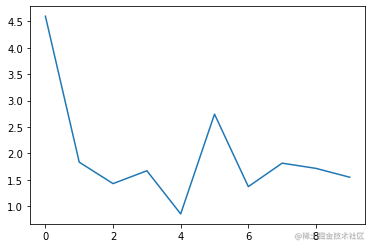# 动手写一个深度学习框架(1) 用 pytorch 搭建神经网络### 小目标### 参考资料

• George Hotz 分享关于 tinygrad 分享
• pytorch 官方文档### 基本要求

• 了解深度学习一般知识
• 熟悉 python 编程语言
• 精通 numpy、matplotlab 等 python 主流库
• 熟悉 pytorch### 准备工作

%pylab inline
import numpy as np
from tqdm import trange
np.set_printoptions(suppress=True)
import torch
import torch.nn as nn
# torch.set_printoptions(precision=2)
torch.set_printoptions(sci_mode=False)

## 准备数据集

def fetch(url):
import requests, gzip, os, hashlib, numpy
fp = os.path.join("/tmp", hashlib.md5(url.encode('utf-8')).hexdigest())
if os.path.isfile(fp):
with open(fp, "rb") as f:
else:
with open(fp, "wb") as f:
dat = requests.get(url).content
f.write(dat)
return numpy.frombuffer(gzip.decompress(dat), dtype=np.uint8).copy()
X_train = fetch("http://yann.lecun.com/exdb/mnist/train-images-idx3-ubyte.gz")[0x10:].reshape((-1, 28, 28))
Y_train = fetch("http://yann.lecun.com/exdb/mnist/train-labels-idx1-ubyte.gz")[8:]
X_test = fetch("http://yann.lecun.com/exdb/mnist/t10k-images-idx3-ubyte.gz")[0x10:].reshape((-1, 28, 28))
Y_test = fetch("http://yann.lecun.com/exdb/mnist/t10k-labels-idx1-ubyte.gz")[8:]X_train.shape #(60000, 28, 28)

imshow(X_train,cmap='gray')## 定义模型class ANet(torch.nn.Module):
def __init__(self):
super(ANet,self).__init__()
self.l1 = nn.Linear(784,128)
self.act = nn.ReLU()
self.l2 = nn.Linear(128,10)
def forward(self,x):
x = self.l1(x)
x = self.act(x)
x = self.l2(x)
return x
model = ANet()

• 最后两个维度进行展平输入维度(m,784)
• 类型为 pytorch 提供的 tensor 类型，数值类型为浮点类型的数据
model(torch.tensor(X_train[0:10].reshape((-1,28*28))).float())

epochs = 10
tbar = trange(epochs)
for i in tbar:
tbar.set_description(f"iterate {i}\n")

epochs = 10
batch_size = 32
tbar = trange(epochs)
for i in tbar:
samp = np.random.randint(0,X_train.shape,size=(batch_size))
print(samp)

## 训练epochs = 10
batch_size = 32
tbar = trange(epochs)
# 定义损失函数，损失函数使用交叉熵损失函数
loss_fn = nn.CrossEntropyLoss()
# 定义优化器
for i in (t:=trange(epochs)):
#对数据集中每次随机抽取批量数据用于训练
samp = np.random.randint(0,X_train.shape,size=(batch_size))
X = torch.tensor(X_train[samp].reshape((-1,28*28))).float()
Y = torch.tensor(Y_train[samp]).long()
# 将梯度初始化

# 模型输出
out = model(X)
#计算损失值
loss = loss_fn(out,Y)
# 计算梯度
loss.backward()
# 更新梯度
optim.step()
t.set_description(f"loss {loss.item():0.2f}")

#### 定义损失函数

$\frac{\exp(x_i)}{\sum_{j=1}^n \exp(x_j)}$

$l=(x,y) = L\{l_1,\cdots,l_N\}^T$
$l_n = -w_{y_n} \frac{\exp(x_{n,y_n})}{\sum_{c=1}^C \exp(x_{n,c})}$
epochs = 10
batch_size = 32
tbar = trange(epochs)
# 定义损失函数，损失函数使用交叉熵损失函数
loss_fn = nn.CrossEntropyLoss()
# 定义优化器

losses,accs = [],[]

for i in (t:=trange(epochs)):
#对数据集中每次随机抽取批量数据用于训练
samp = np.random.randint(0,X_train.shape,size=(batch_size))
X = torch.tensor(X_train[samp].reshape((-1,28*28))).float()
Y = torch.tensor(Y_train[samp]).long()
# 将梯度初始化

# 模型输出
out = model(X)
#计算准确度
pred = torch.argmax(out,dim=1)
acc = (pred == Y).float().mean()

#计算损失值
loss = loss_fn(out,Y)
# 计算梯度
loss.backward()
# 更新梯度
optim.step()
#
loss, acc = loss.item(),acc.item()
losses.append(loss)
accs.append(acc)
t.set_description(f"loss:{loss:0.2f}, acc: {acc:0.2f}")

plot(losses)